# ACT Math : How to find the perimeter of a 45/45/90 right isosceles triangle

## Example Questions

### Example Question #9 : Isosceles Triangles

What is the perimeter of an isosceles right triangle with an hypotenuse of length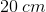?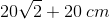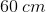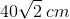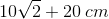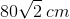Explanation:

Your right triangle is a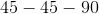triangle. It thus looks like this: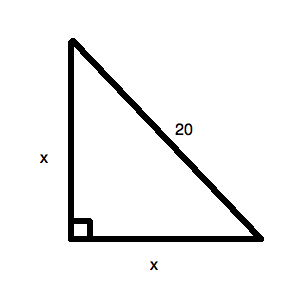Now, you know that you also have a reference triangle fortriangles. This is: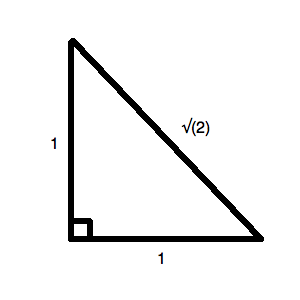This means that you can set up a ratio to find. It would be: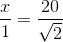Your triangle thus could be drawn like this: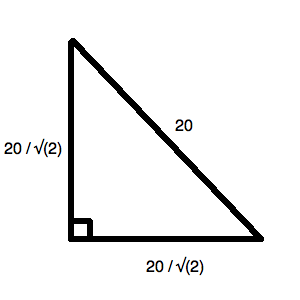Now, notice that you can rationalize the denominator of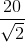: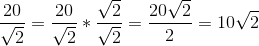Thus, the perimeter of your figure is: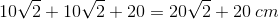### Example Question #10 : Isosceles Triangles

What is the perimeter of an isosceles right triangle with an area of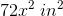?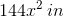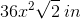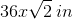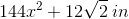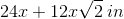Explanation:

Recall that an isosceles right triangle is also atriangle. Your reference figure for such a shape is:or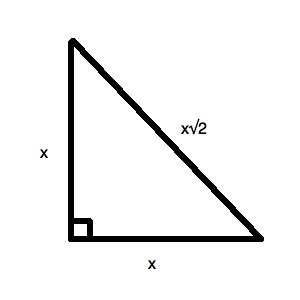Now, you know that the area of a triangle is: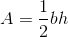For this triangle, though, the base and height are the same. So it is: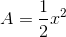Now, we have to be careful, given that our area contains. Let's use, for "side length":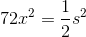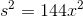Thus,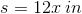. Now based on the reference figure above, you can easily see that your triangle is: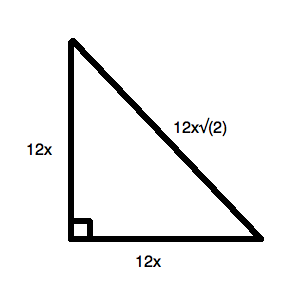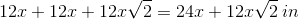### Example Question #1 : How To Find The Perimeter Of A 45/45/90 Right Isosceles Triangle

A tree isfeet tall and is planted in the center of a circular bed with a radius offeet. If you want to stabalize the tree with ropes going from its midpoint to the border of the bed, how long will each rope measure?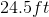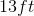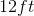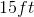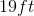Explanation:

This is a right triangle where the rope is the hypotenuse. One leg is the radius of the circle, 5 feet. The other leg is half of the tree's height, 12 feet. We can now use the Pythagorean Theoremgiving us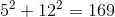. If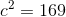then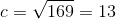.

### Example Question #2 : How To Find The Perimeter Of A 45/45/90 Right Isosceles Triangle

An isosceles right triangle has a hypotenuse of length. What is the perimeter of this triangle, in terms of?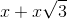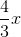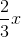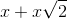Explanation:

The ratio of sides to hypotenuse of an isosceles right triangle is always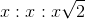. With this in mind, settingas our hypotenuse means we must have leg lengths equal to: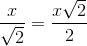Since the perimeter has two of these legs, we just need to multiply this byand add the result to our hypothesis: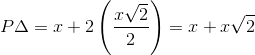So, our perimeter in terms ofis: